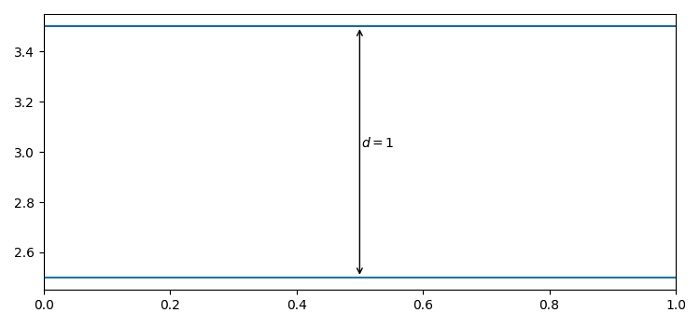# Plotting distance arrows in technical drawing in Matplotlib

To plot distance arrows in technical drawing in matplotlib, we can use annotate() method with arrow properties.

## Steps

• Set the figure size and adjust the padding between and around the subplots.

• Add a horizontal line across the axis using axhline() method, i.e., y=3.5.

• Add a horizontal line across the axis using axhline() method, i.e., y=2.5.

• Use annotate() method to draw an arrow line to show the distance and in the very next statement, use annotate() method again to display the distance between two horizontal lines.

• To display the figure, use show() method.

## Example

from matplotlib import pyplot as plt
plt.rcParams["figure.figsize"] = [7.50, 3.50]
plt.rcParams["figure.autolayout"] = True
plt.axhline(3.5)
plt.axhline(2.5)
plt.annotate(
'', xy=(0.5, 3.5), xycoords='data',
xytext=(0.5, 2.5), textcoords='data',
arrowprops={'arrowstyle': '<->'})
plt.annotate(
'$\it{d=1}$', xy=(0.501, 3.0), xycoords='data',
xytext=(0.5, 3.5), textcoords='offset points')
plt.show()

## Output